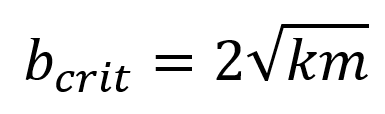Initial Conditions:

Critical Damping: A Bumpy Road

How do you tune shock absorbers to best deal with a bumpy road? Remember critical damping, b2 = 4mk, is the smallest damping for which oscillations don't occur. That might seem the best choice, but in fact slightly smaller damping, with a little oscillation, has a faster response. We plot two curves corresponding to b = 0.5, the critical value for the k, m values we've given, and b = 0.4. We plot the areas under the two curves (counting area below as positive as well) to give some idea of the amount of deviation from equilibrium. The area is less for the subcritical value. In fact, it's almost the same for b = 0.2, but then looking at the curve it's not clear that this is a comfortable solution! We also allow the option of starting at a given displacement at zero velocity (the default) and starting at the equilibrium position but with some velocity (a sudden kick). For that situation, it seems critical value might be a little better. Both scenarios no doubt occur in a bumpy car ride. Check out the walk-through below to learn more about critical damping of oscillators.

Walk-Through

How do you think this will change for m = 0.5 and k = 0.5?

Try it! Then try both equal to 1. How, exactly, has the critical damping changed?

What happens at m = 1 and k = 0.25?  Check it out.  How does this critical damping differ from m = 0.5 and k = 0.5? Or does it?

Here's the relevant lecture.

Program by: Carter Hedinger

1.000.50